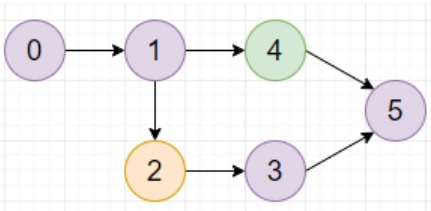# Program to find largest color value in a directed graph in Python

PythonServer Side ProgrammingProgramming

#### Beyond Basic Programming - Intermediate Python

Most Popular

36 Lectures 3 hours

#### Practical Machine Learning using Python

Best Seller

91 Lectures 23.5 hours

#### Practical Data Science using Python

22 Lectures 6 hours

Suppose we have a directed graph with n colored nodes and m different edges. And the nodes are numbered from 0 to n-1. We have a string col with lowercase letters, where col[i] represents the color of the ith node in this graph (0-indexed). We also have an edge list where edges[j] = (u, v) represents, there is an edge between u and v.

A valid path in the graph is a sequence of nodes xi for all i from 1 to k, such that there is a directed edge from xi to xi+1. The color of the path is the most frequent node color of that path. We have to find largest color value of any valid path in that graph. If there is a cycle in the graph then return -1.

So, if the input is like col = "aabada" edges = [(0,1),(1,4),(1,2),(2,3),(3,5),(4,5)],then the output will be 4 as 0 -> 1 -> 2 -> 3 -> 5 has longest path with color 'a'.

To solve this, we will follow these steps −

• n:= size of col

• graph:= given graph from the edge list

• indegree:= map containing nodes and their in-degree values

• queue:= a new list

• dp:= make an array of size n x 26, and fill with 0

• colorvalues:= make a list with order of c in alphabet for all c in col

• for u in range 0 to n - 1, do

• if u is not in indegree, then

• insert u at the end of queue

• dp[u, colorvalues[u]]:= 1

• visited:= 0

• while queue is not empty, do

• u:= front element of queue and delete it after

• visited := visited + 1

• for each v in graph[u], do

• for c in range 0 to 25, do

• dp[v, c] = maximum of dp[v, c] and (dp[u, c] + (1 if c is same as colorvalues[v], otherwise 0)

• indegree[v] := indegree[v] - 1

• if indegree[v] is same as 0, then

• insert v at the end of queue

• del indegree[v]

• if visited < n, then

• return -1

• return maximum element in dp

## Example

Let us see the following implementation to get better understanding

from collections import defaultdict
def solve(col, edges):
n=len(col)
graph=defaultdict(list)
indegree=defaultdict(int)

for u,v in edges:
graph[u].append(v)
indegree[v]+=1

queue=[]
dp=[*26 for _ in range(n)]
colorvalues=[ord(c)-ord("a") for c in col]
for u in range(n):
if u not in indegree:
queue.append(u)
dp[u][colorvalues[u]]=1

visited=0
while queue:
u=queue.pop()
visited+=1

for v in graph[u]:
for c in range(26):
dp[v][c]=max(dp[v][c],dp[u][c] + (c==colorvalues[v]))
indegree[v]-=1
if indegree[v]==0:
queue.append(v)
del indegree[v]
if visited<n:
return -1
return max(max(x) for x in dp)

print(solve(col, edges))
"aabada", [(0,1),(1,4),(1,2),(2,3),(3,5),(4,5)]

4